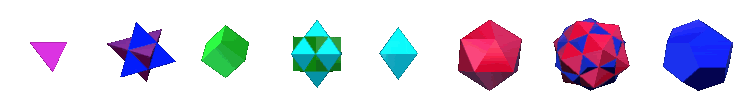PlatonicSolids.info

National Standards

National Council of Teachers of Mathematics (NCTM)

Geometry Standard Instructional programs from prekindergarten through grade 12 should enable all students to—

Analyze characteristics and properties of two- and three-dimensional geometric shapes and develop mathematical arguments about geometric relationships

 Pre-K–2 Expectations: In prekindergarten through grade 2 all students should–
 • recognize, name, build, draw, compare, and sort two- and three-dimensional shapes; • describe attributes and parts of two- and three-dimensional shapes; • investigate and predict the results of putting together and taking apart two- and three-dimensional shapes.
 • identify, compare, and analyze attributes of two- and three-dimensional shapes and develop vocabulary to describe the attributes; • classify two- and three-dimensional shapes according to their properties and develop definitions of classes of shapes such as triangles and pyramids; • investigate, describe, and reason about the results of subdividing, combining, and transforming shapes; • make and test conjectures about geometric properties and relationships and develop logical arguments to justify conclusions.
 • precisely describe, classify, and understand relationships among types of two- and three-dimensional objects using their defining properties; • understand relationships among the angles, side lengths, perimeters, areas, and volumes of similar objects; • create and critique inductive and deductive arguments concerning geometric ideas and relationships, such as congruence, similarity, and the Pythagorean relationship.
 • analyze properties and determine attributes of two- and three-dimensional objects; • explore relationships (including congruence and similarity) among classes of two- and three-dimensional geometric objects, make and test conjectures about them, and solve problems involving them; • establish the validity of geometric conjectures using deduction, prove theorems, and critique arguments made by others; • use trigonometric relationships to determine lengths and angle measures.

Specify locations and describe spatial relationships using coordinate geometry and other representational systems

 Pre-K–2 Expectations: In prekindergarten through grade 2 all students should–
 • describe, name, and interpret relative positions in space and apply ideas about relative position; • describe, name, and interpret direction and distance in navigating space and apply ideas about direction and distance;
 • describe location and movement using common language and geometric vocabulary;
 • use coordinate geometry to represent and examine the properties of geometric shapes; • use coordinate geometry to examine special geometric shapes, such as regular polygons or those with pairs of parallel or perpendicular sides.
 • investigate conjectures and solve problems involving two- and three-dimensional objects represented with Cartesian coordinates.

Apply transformations and use symmetry to analyze mathematical situations

 Pre-K–2 Expectations: In prekindergarten through grade 2 all students should–
 • recognize and apply slides, flips, and turns; • recognize and create shapes that have symmetry.
 • predict and describe the results of sliding, flipping, and turning two-dimensional shapes; • describe a motion or a series of motions that will show that two shapes are congruent; • identify and describe line and rotational symmetry in two- and three-dimensional shapes and designs.
 • describe sizes, positions, and orientations of shapes under informal transformations such as flips, turns, slides, and scaling; • examine the congruence, similarity, and line or rotational symmetry of objects using transformations.
 • understand and represent translations, reflections, rotations, and dilations of objects in the plane by using sketches, coordinates, vectors, function notation, and matrices; • use various representations to help understand the effects of simple transformations and their compositions.

Use visualization, spatial reasoning, and geometric modeling to solve problems

 Pre-K–2 Expectations: In prekindergarten through grade 2 all students should–
 • create mental images of geometric shapes using spatial memory and spatial visualization; • recognize and represent shapes from different perspectives; • relate ideas in geometry to ideas in number and measurement; • recognize geometric shapes and structures in the environment and specify their location.
 • build and draw geometric objects; • create and describe mental images of objects, patterns, and paths; • identify and build a three-dimensional object from two-dimensional representations of that object; • identify and draw a two-dimensional representation of a three-dimensional object; • use geometric models to solve problems in other areas of mathematics, such as number and measurement; • recognize geometric ideas and relationships and apply them to other disciplines and to problems that arise in the classroom or in everyday life.
 • draw geometric objects with specified properties, such as side lengths or angle measures; • use two-dimensional representations of three-dimensional objects to visualize and solve problems such as those involving surface area and volume; • use visual tools such as networks to represent and solve problems; • use geometric models to represent and explain numerical and algebraic relationships; • recognize and apply geometric ideas and relationships in areas outside the mathematics classroom, such as art, science, and everyday life.
 • draw and construct representations of two- and three-dimensional geometric objects using a variety of tools; • visualize three-dimensional objects and spaces from different perspectives and analyze their cross sections; • use vertex-edge graphs to model and solve problems; • use geometric models to gain insights into, and answer questions in, other areas of mathematics; • use geometric ideas to solve problems in, and gain insights into, other disciplines and other areas of interest such as art and architecture.

These Standards come from the NCTM website.

Also see the NCTM Focal Points.

The search engine below is customized to take you only to geometry sites.

home - video - book - merchandise - discussion group - wallpaper - images - animated GIFs

origami - paper polyhedra - screenplay - lesson plans - standards - links - books - bio - contact - RSS - iTunes

This site and all of its contents were created by Dan Radin unless otherwise stated.